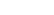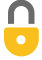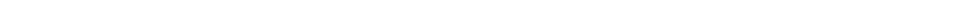Problem 60
Q

# If a car generates 18 hp when traveling at a steady 95 km/h, what must be the average force exerted on the car due to friction and air resistance?A
$510\textrm{ N}$In order to watch this solution you need to have a subscription.VIDEO TRANSCRIPT

This is Giancoli Answers with Mr. Dychko. In order for this car to go at constant speed, the force that its engine applies, you know, to the wheels, to the ground and so on, that force due to the car, one-direction, has to equal the force of the, you know, air resistance and so on and friction go in the opposite direction; those are in equal in magnitude. So the power exerted by the engine of the car is equal to the force that the car applies to the ground which then, in turn, applies the same force back to the car. Anyway, let me try to not be too complicated here. So we have force of the car times its speed equals the power; that's average velocity and average power. So, we'll divide both sides by v here, divide by average velocity, and we get that the force exerted by the air and the friction combine, which is the same as a force exerted by the car, as you can see in this diagram, they are equal length arrows. That's going to be the power divided by the speed. So that's 18 horsepower times 746 watts per horsepower; let's convert this into mks units—meters, kilograms and seconds— that's the usual routine for any formula converted into those units, and divided by the velocity, which will convert it into meters and seconds as well. So 95 kilometers per hour times 1 hour for every 3600 seconds and then times by 1000 meters per kilometer gives us meters per second on the bottom. And, if you look over in the calculator, you'll see how that's punched into the calculator and you end up with about 510 newtons of force.By anochc on Sun, 10/19/2014 - 6:14 AM

please i need for questions 83 up to 94 pages to complete my homework assignment
thanks
ebe

By Mr. Dychko on Mon, 10/20/2014 - 4:37 PM

Hi anochc, since it would be enormously time consuming to also answer the "General Problems", I'm limiting coverage only to the regular "Problems", of which there are still more than 1700. I hope you understand. My suggestion would be to try and find a regular "Problem" that is similar to the "General Problems" you're asking about, and see if you can apply the same problem solving technique.

Cheers,
Mr. Dychko

Giancoli Answers, including solutions and videos, is copyright © 2009-2023 Shaun Dychko, Vancouver, BC, Canada. Giancoli Answers is not affiliated with the textbook publisher. Book covers, titles, and author names appear for reference purposes only and are the property of their respective owners. Giancoli Answers is your best source for the 7th and 6th edition Giancoli physics solutions.